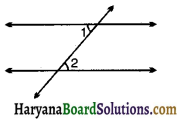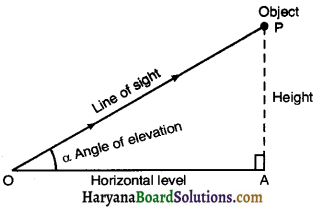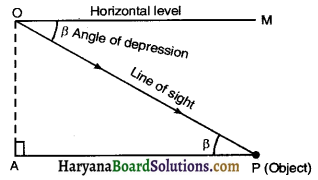# HBSE 10th Class Maths Notes Chapter 9 Some Applications of Trigonometry

Haryana State Board HBSE 10th Class Maths Notes Chapter 9 Some Applications of Trigonometry Notes.

## Haryana Board 10th Class Maths Notes Chapter 9 Some Applications of Trigonometry

Introduction
We have studied about trigonometric ratios in earlier classes. In this chapter, we shall use the trigonometric ratios, to solve the problems regarding the heights and distances of various objects. Two common terms used in this chapter are angle of elevation and angle of depression.
1. Elevation: The height to which something is raised above a point of reference.
2. Depression: The depth to which something is lowered below a point of reference.
3. Line of sight: The line drawn from the eye of an observer to the point in the object viewed by the observer.
4. Complementary angles: Two angles having a sum of 90°, are called complementary angles.5. Speed = Distance/Time
6. Alternate interior angle: The pair of angles on opposite sides of the transversal but inside the two lines are called alternate interior angles.
If the two lines are parallel, then the alternate interior angles formed are equal.Heights and Distances
Angle of Elevation: If a person is looking up at an object, the acute angle measured from the horizontal level to the line of sight when the object being viewed is called the angle of elevation. HereO is the point of observation, P is the position of the object, OP is the line of sight. OA is horizontal level and α is the angle of elevation.

Angle of Depression: The angle between the line of sight and horizontal level through the eye of the observer, when the object being viewed is below the horizontal level is called angle of depression. Here position of observer is at O. OM is the horizontal level through O. OP is the line of sight. β is the angle of depression when the object at P is observed from O.Remarks:
(a) Numerically the angle of elevation is equal to the angle of depression.
(b) The angle of elevation and angle of depression both are measured with the horizontal.
(c) The angle of elevation or depression increases as the observer moves towards the object.